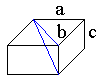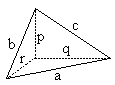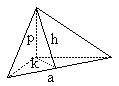# Analogues and Generalizations of the Pythagorean Theorem

Pythagorean Theorem is one of the most fundamental results of Mathematics. Using the theorem we define what's known as euclidean distance dist2. This notion of distance extends to spaces with scalar product - Hilbert spaces.

Proofs ##13, 17, and 18 gave us plane generalizations of the theorem. Below I consider an analogue that holds in the $3$-dimensional space $\mathbb{R}^{3}.$ The statement leads to the definition of euclidean distance in $\mathbb{R}^{3}.$ Afterwards, there is an additional and unexpected analog of the theorem in $\mathbb{R}^{3}.$It's convenient to think of the Pythagorean Theorem as defining the length of the diagonal in a rectangle when its two sides are given. Now consider a parallelepiped with sides $a,$ $b,$ and $c.$ Incidently, the diagonal in question serves as the hypotenuse of the right triangle formed by the edge $c$ and the diagonal of the face $ab.$ The latter, by the Pythagorean Theorem, equals $\sqrt{a^{2}+b^{2}}.$ Applying it the second time gives the length of the diagonal as $\sqrt{a^{2}+b^{2}+c^{2}}.$

When we moved from a $2$-dimensional space to a $3$-dimensional space the formula for the diagonal of a shape built on orthogonal segments remained virtually the same except the number of terms grew from $2$ to $3,$ as appropriate. However, in both cases squared were line segments. Pythagorean Theorem has an analog where squared are areas of triangles.The theorem applies to a special kind of tetrahedra in which all three edges emanating from one of the vertices are perpendicular to each other. One can obtain such a pyramid by cutting a corner from a parallelepiped. Let's introduce the areas $A,B,C$ of the faces that house right angles, and let $D$ be the area of the remaining face. We have

$A = qr/2,$ $B = rp/2,$ $C = pq/2.$

What I want to show is that $A^{2} + B^{2} + C^{2} = D^{2}.$Draw a plane through $p$ perpendicular to $a.$ Then both $k$ and $h$ will be perpendicular to $a.$ We find that $D = ha/2,$ while $h^{2} = k^{2} + p^{2}.$ Also $A = ak/2.$ Therefore,

\begin{align} 4D^{2} &= a^{2}h^{2}\\ &= a^{2}(k^{2} + p^{2})\\ &= 4A^{2} + a^{2}p^{2}\\ &= 4A^{2} + (r^{2} + q^{2})p^{2}\\ &= 4A^{2} + (rp)^{2} + (pq)^{2}\\ &= 4A^{2} + 4B^{2} + 4C^{2} \end{align}

Q.E.D.Oops, I almost forgot the Cosine Law which is a clear generalization of the Pythagorean Theorem. For a triangle with sides $a,$ $b,$ and $c$ and angle $C$ opposite the side $c,$ one has

$c^{2} = a^{2} + b^{2} - 2ab\cdot \cos(C)$

which, in turn, admits a generalization to higher dimensional spaces.

The fact expressed nowadays by the single identity appears in Euclid's Elements as two separate propositions: II.12 for obtuse-angled triangles and II.13 for the acute ones.

Dr. Scott Brodie from the Mount Sinai School of Medicine, NY, sent me a proof of the theorem and a dynamic Geometer's SketchPad illustration.

### References

1. G. Polya, Mathematical Discovery, John Wiley and Sons, 1981.